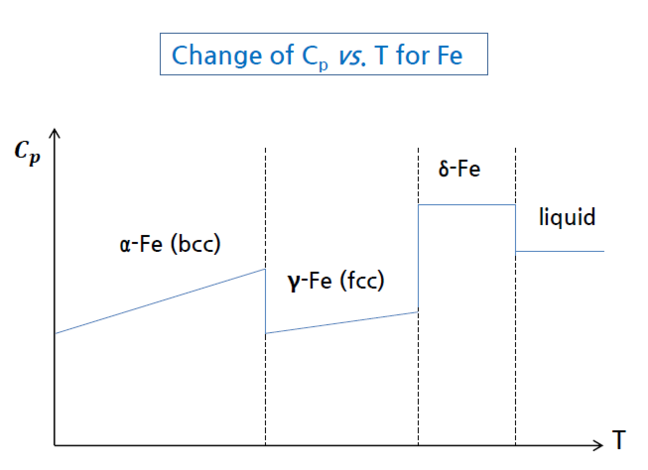1.13

# T_ P dependence of heat capacity

Heat capacity

#### ♦  Temperature dependence of heat capacity

• $C$$p$ usually give as $C$$p$=$a$+$bT$+$cT$$2$+$dT$$2$
• When phase changes → Heat capacity changes abruptly#### ♦  Pressure dependence of heat capacity

• $C$$p$ of ideal gas
For ideal gas, $C$$p$ is indenepdne of pressure. Each molecule behaves        independently.

• $C$$p$ of solid
In most cases, $C$$p$ is a function of temperature and not a function of        pressure, but at high pressire, $C$$p$ is strongly dependent on pressure.